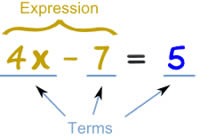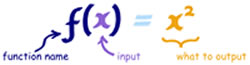# Difference between Equations and Functions

##### Key difference: In mathematics, an equation is used to denote the equality between two expressions. A function, on the other hand, is in much more complex than an equation. A function is used to denote a relation between a set of inputs and a set of corresponding outputs.An equation and a function are two of the basic foundations of algebra, a subject matter under mathematics. Both can be quite complex in nature, however, in their basic form they may be confused as each other. The main difference between an equation and a function is the fact that an equation usually deals with a single input, whereas, a function can have numerous inputs.

In mathematics, an equation is used to denote the equality between two expressions. Essentially, an equation is written as an expression equals to another expression. For example: x + 2 = 5. This denotes that whatever is x, if you add 2 to it, will be equal to 5. Hence, we can solve the equation for x, which is 3, as 3 + 2 = 5.

Equations can be more complex than that and can include more than one variables, such as x, y, z, etc. in a single equation. For example: 3x + 2y – z = 4. However, each alphabet will correspond to one number. In this case, x = 1, y = 2 and z = 3.

Hence,

3x + 2y – z = 4 becomes

3(1) + 2(2) – 3 = 4 which is

3 + 4 – 3 = 4 essentially

4 = 4

A function, on the other hand, is in much more complex than an equation. A function is used to denote a relation between a set of inputs and a set of corresponding outputs. Essentially, an input should give a single output. A function is a relationship between two variables. For example: f(x) = x + 2. As per this function, whatever, the input is, it will give you a single output, which will be the input plus 2. Let’s solve this function:

 Input Function Output x f(x) = x + 2 f(x) 1 1 + 2 3 2 2 + 2 4 3 3 + 2 5 4 4 + 2 6 5 5 + 2 7

And so on…Functions have various applications in mathematics, physics, computer science, etc. They can range from a simple function, as denoted above, to complex formulas or algorithms. A function can be thought of as a machine that gives an output for an input, and that output is somehow related to the input.

A function always has three parts: the input, the relationship, and the output. The classic way of writing a function is with "f(x) = ... ", where x denotes the input and f(x) denotes the output.

As stated above, the main difference between an equation and a function is that an equation usually has only one input that will give lead to the expressions being equal. Whereas, a function has various inputs, each of which will give a output.

Image Courtesy: mathsisfun.com

After going through many websites and videos, I was finally able to get my head around this concept because of your excellent explanation. Thank u so much

I particularly appreciated the story of your struggle to get to this point. I was affirmed in my tendency to bulldog to the bottom of things until they finally become clear. Have to choose our battles, though, don't we. And keeping a neat office isn't one of them! THAT I really understand. Oh, for more time. Jim Croce said it well: there never seems to be enough time to do the things that you want to do once you find them....

The best explanation i've ever heard.

thnx

Very helpful in explaining to my daughter.

Nice explanation I understood easily

it is very helpful for me